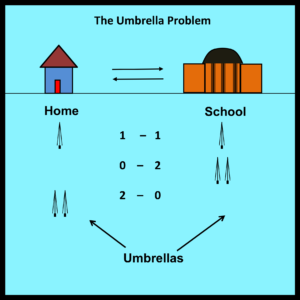# Exercises, Puzzles, etc.

Exercises

1. The Umbrella problem discussed in Sections 5.7 – 5.9 can be extended in a number of ways.   The simplest extensions involve increasing the number of umbrellas at the start of the semester from two to three or four. How does this affect the proportion of wet trips? A more challenging problem is to express the proportion of wet trips as a function of N, where N represents the number of umbrellas.

[For the next few problems, return to the original case where the number of umbrellas is equal to two.]1. As noted in the text, the professor never gets wet if it rains all the time since the professor simply carries the same umbrella back and forth each day. Similarly, the professor never gets wet if it never rains at all (clearly – no umbrella is needed in this case). This suggests that there is a value for the proportion of rainy trips that will maximize the number of times the professor gets wet. What is that proportion? Can this proportion be derived without using calculus?  If not, can it at least be shown (without using calculus) that this proportion is not equal to one half?

1. What about extending the analysis to trajectories whose endpoints are not matched? The professor always has one available umbrella at the start of the semester. What does the solution look like when the number of available umbrellas at the end of the semester is equal to zero … or two? How large is the error in these cases if the simplified solution (with matched endpoints) is assumed to be correct? Under what conditions does this error approach zero?

1. The solution in Section 5.7 depends on the assumption that the proportion of rainy trips is empirically independent of the number of available umbrellas at the start of the trip. Suppose this is not true.   What does the solution look like in such cases? How large is the error in these cases if the simplified solution (with empirical independence) is assumed to be correct? For example, suppose all three proportions are within 10% of each other. How large can the error possibly be in this case?